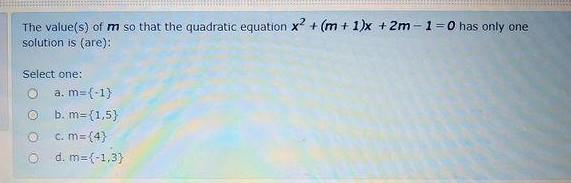### Create an Account

Home / Questions / The value(s) of m so that the quadratic equation X² + (m + 1)x + 2m-1-0 has only one solut...

# The value(s) of m so that the quadratic equation X² + (m + 1)x + 2m-1-0 has only one solution is (are): Select one: a. m=(-1) b. m={1,5} C. m={4} d. m={-1,3)

The value(s) of m so that the quadratic equation X² + (m + 1)x + 2m-1-0 has only one solution is (are): Select one: a. m=(-1) b. m={1,5} C. m={4} d. m={-1,3)May 29 2021 View more View LessSubscribe To Get Solution